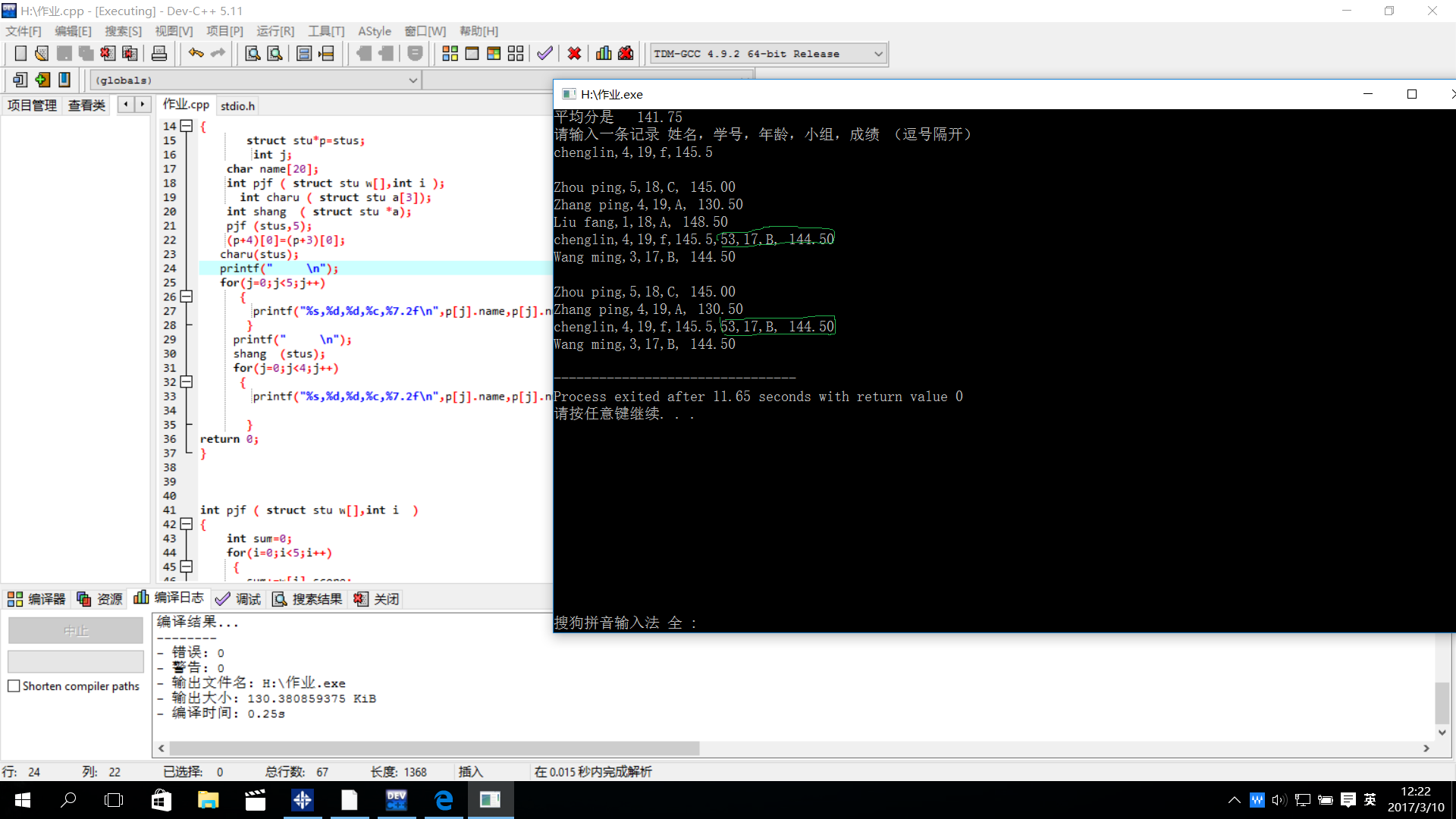###### 名叫黑客的技术小白

2017-03-10 04:28 阅读 895

# c语言结构体数组赋值新值但旧值还在

#include
#include
struct stu{
char name;

int num;

int age;

char group;

float score;

}stus = {{"Zhou ping", 5, 18, 'C', 145.0},
{"Zhang ping", 4, 19, 'A', 130.5},
{"Liu fang", 1, 18, 'A', 148.5},
{"Wang ming", 3, 17, 'B', 144.5} } ;
int main ()
{

struct stu*p=stus;
int j;
char name;
int pjf ( struct stu w[],int i );
int charu ( struct stu a);
int shang ( struct stu *a);
pjf (stus,5);
(p+4)=(p+3);
charu(stus);
printf(" \n");
for(j=0;j<5;j++)
{
printf("%s,%d,%d,%c,%7.2f\n",p[j].name,p[j].num,p[j].age,p[j].group,p[j].score);
}
printf(" \n");
shang (stus);
for(j=0;j<4;j++)
{
printf("%s,%d,%d,%c,%7.2f\n",p[j].name,p[j].num,p[j].age,p[j].group,p[j].score);

``````   }
``````

return 0;
}

int pjf ( struct stu w[],int i )
{
int sum=0;
for(i=0;i<5;i++)
{
sum+=w[i].score;
}
printf ("平均分是 %7.2f\n",sum/4.0);
return 0;
}

int charu ( struct stu a)
{
printf("请输入一条记录 姓名，学号，年龄，小组，成绩 （逗号隔开）\n");
scanf("%s,%d,%d,%c,%f",a.name,a.num,a.age,a.group,a.score);
return 0;
}

int shang ( struct stu *a)
{
(a+2)=(a+3);
(a+3)=(a+4);
return 0;
}• 点赞
• 写回答
• 关注问题
• 收藏
• 复制链接分享

#### 3条回答默认 最新

• 已采纳

第一次看见解引这么玩的哈哈
问题一：pjf这个函数的i的意义是什么，怎么在for的时候又给i重新赋了个值。
问题二：初始化的时候只有4个元素，但是在pjf这个函数里面怎么访问到了第五个元素的位置，这个虽然是可以的，而且系统自动帮你初始化了。
问题三：也就是最致命的，你在输入的时候只是把name那一项当作字符串了，期间没有制表符，所以此时你输入的所有东西都是name，不是其他的。
之后还有一些其他地方的小问题，加油

点赞 评论 复制链接分享
• 各人觉得是在调用插入子函数的那里出现错误，其他地方都没问题，题主再想想。

点赞 评论 复制链接分享
• 楼主要是知道错误了，告诉我哦，楼主也是对指针掌握得不好。

点赞 评论 复制链接分享